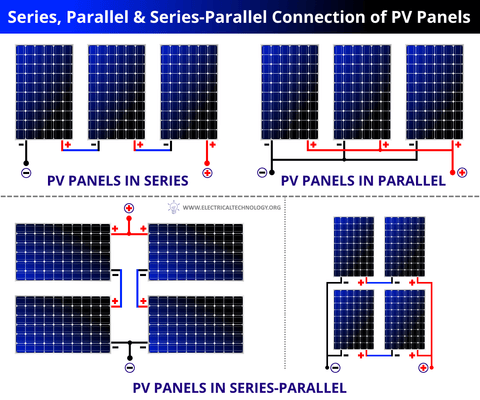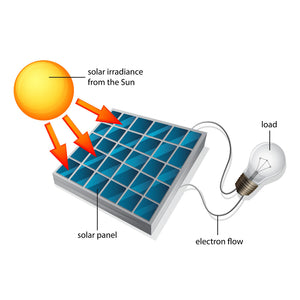# Intro to Solar Energy

Basic Solar Cell Information and Graphs

Sunlight contains many colors of light which are received as wavelengths. Color and relative power in each color of light is determined by the measure of these wavelengths. Figure 1 below plots this relationship between wavelengths and color among the spectral distribution of ordinary light.Figure 1: Wavelength to Power Graph

The relative response of a typical silicon solar cell across the light spectrum is represented in Figure 2. As we can see from the chart, silicon solar cells have a high response over a broad range of wavelengths.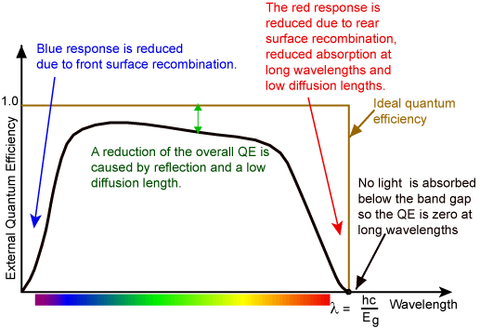Figure 2: Wavelength to Solar Cell Response Graph

In most circumstances, solar cells are not exposed to maximum levels of sunlight. Figure 3 shows the resulting output of a solar cell when exposed to different levels of sunlight intensity. Notice that the terminal voltage is not significantly effected by the sunlight intensity.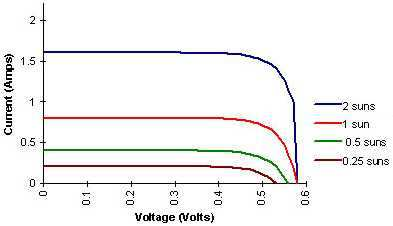Figure 3: Wavelength to Solar Cell Response Graph

The average number of peak sun hours per day varies from one area of the country to another. Figure 4 shows the yearly average peak sun hours and in turn the potential for solar energy applications for different parts of the country.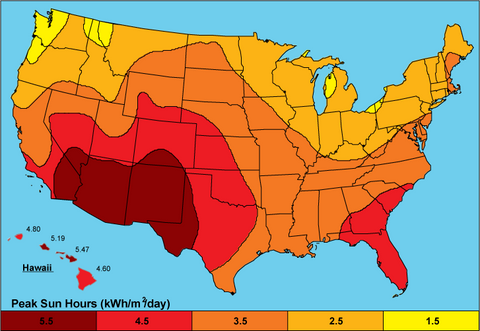Figure 4: Average Peak Sun Hours/Day

Series and Parallel Connections

In order to transmit the electricity of the sunlight activated "N-P" junction of the solar cell, simply attach a conductor (copper wire) to each side of the solar cell. The resulting electrical current is determined by the surface area of the cell, with each cell producing one-half volt. In order to vary the amount of current of voltage produced, solar cells can be connected in series and/or parallel.

Series:

Solar cells connected in series (- to + to - to +...) will increase the voltage output while the current remains the same.Parallel:

Solar cells connected in parallel (- to - and + to +) will increase the current output while the voltage remains the same.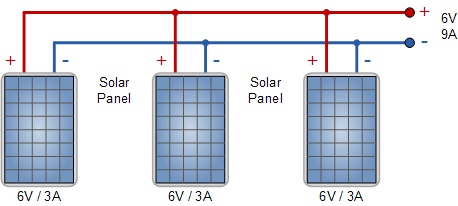Both:

By combining these two principles one can increase both the current and voltage output of a solar cell system.